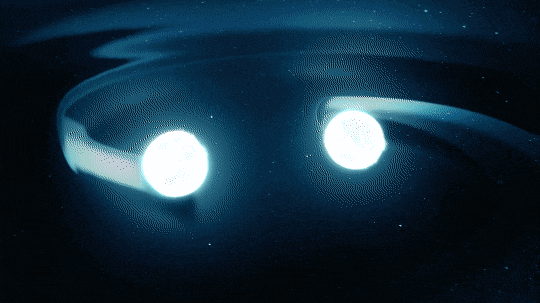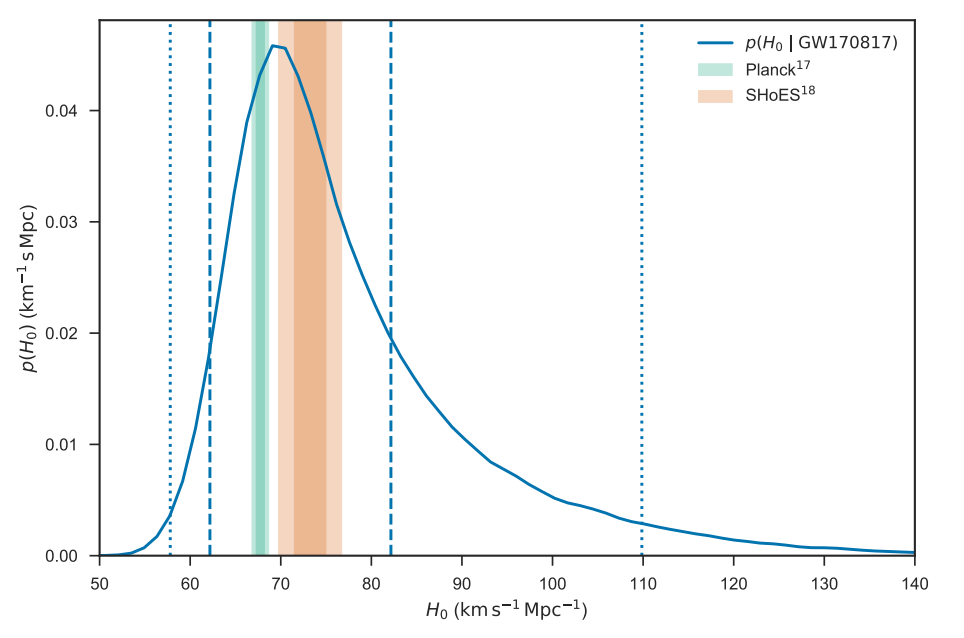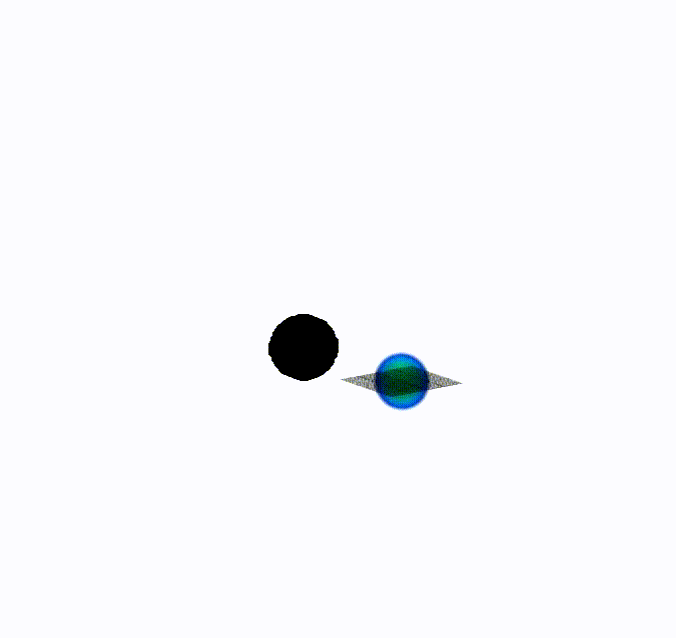# Ideal cosmic yardstick: neutron star black hole mergers as standard sirens

Authors: Salvatore Vitale, Hsin-Yu Chen

First Author’s Institution: Massachusetts Institute of Technology, Massachusetts, USA

Status: Physical Review Letters, open access on arXiv

A little over a year ago, on the 17 August, LIGO (Laser Interferometer Gravitational Wave Observatory) detected a binary neutron star merger (known as GW170817). This was groundbreaking for a number of reasons. First of all, it was a multi-messenger detection – meaning we observed ripples in spacetime (gravitational waves) traveling through the LIGO detector and, around two seconds later, saw light across the electromagnetic spectrum from the same collision.

In addition, because we detected the collision through two different physical mechanisms (light and gravitation) it was possible to combine these pieces of information to get a new estimate for the Hubble constant. The Hubble constant is an extremely important number in cosmology – it describes how fast Universe is expanding and is needed to determine the age and size of our universe. Gravitational wave events with coincident electromagnetic signals are new yardsticks for measuring our universe.

But binary neutron star mergers are not the only type of mergers we expect to see. Neutron star black hole (NSBH) mergers (which are likely to be more rare) could also produce both gravitational and electromagnetic radiation that we could use to deduce the Hubble constant. Indeed, this may be a better method, as is discussed in today’s paper.

### Standard sirens: determining the Hubble constant with gravitational waves from mergers

Hubble’s law is$v=H_0 d$ where v is the velocity of an object receding due to the expansion of the Universe and d is the distance to that object. The Hubble constant$H_0$ relates these two quantities and is estimated to have a value of around 70 (km/s)/Mpc. The calculated value of$H_0$ depends strongly on well-measured distances but measuring distances in our universe can be a tricky business. Direct measurements of astrophysical objects are typically only possible for objects that are close enough to Earth. Otherwise, we use the “cosmic distance ladder“. On the bottom rung of the ladder are direct distance measures like parallax – as you step up the ladder you measure larger distances, with each step up depending on the rungs below it. Therefore, large distances are measured very indirectly, using objects called standard candles. However, calibrating standard candles is challenging, and these uncertainties in calibration affect our estimates of$H_0$.Figure 1: Animation of a binary neutron star collision such as GW170817. Source: https://nasa.tumblr.com/post/166466378849/when-dead-stars-collide

Recently, we have found new, completely independent way of measuring the Hubble constant: mergers (for example, the binary neutron star merger depicted in Figure 1) that produce both gravitational and electromagnetic waves. We call these standard sirens (rather than standard candles) because we estimate the distance to the source using the gravitational wave signal. In addition, we can measure the recessional velocity using the redshift obtained from the electromagnetic signal. For the recent binary neutron star merger (GW170817), we combined these distance and velocity measurements to estimate$H_0 = 70.0 (km/s)/Mpc$. This value sits in between the Planck and SHoEs estimates for$H_0$ and is statistically consistent with both of them (see Figure 2).Figure 2: The blue curve shows the probability of the Hubble constant having the value indicated on the x-axis (based on the GW170817 event), while the green and orange intervals show the Planck and ShoEs predictions respectively. Source: Figure 1 from this LIGO paper.

Other types of mergers could also be electromagnetically brightfor example, neutron star black hole (NSBH) mergers. As the neutron star is tidal disrupted by the black hole, an accretion disk is formed and electromagnetic radiation would be generated (see Figure 3). If we received a gravitational wave from an NSBH merger and also detected it electromagnetically, we could use it to measure$H_0$. On top of that, certain features of this type of merger make it ideal for determining cosmic expansion.Figure 3: Animation of a black hole neutron star merger. Source: http://newscenter.lbl.gov/2017/08/02/new-space-simulations-neutron-star-black-hole-mergers/. Credit: Bryant Garcia.

### Neutron star black hole mergers as the ideal cosmic yardstick

The dominant uncertainty in measuring the Hubble constant using mergers, is in determining the distance to the source using gravitational waves. We need to minimise this uncertainty as much as possible, which we could potentially do by using NSBH mergers instead of binary neutron star mergers. There are two main reasons why NSBH mergers are better for measuring distances compared to binary neutron star mergers.

1. In some cases, it is difficult to tell whether the shape of a particular gravitational wave signal is due to the distance of the source from Earth or due to the orbital inclination angle (i.e. the angle between the line of sight and the total angular momentum of the system). This means a degeneracy exists between these two parameters. NSBH mergers are expected to have significant spin precession, which helps to break this degeneracy.

2. In addition, black holes are heavier than neutron stars, meaning NSBH sources can be observed out to larger distances. Therefore, there is a larger volume in which gravitational events are detectable.

However, NSBH mergers are likely to be more rare than binary neutron star mergersin fact, no NSBH merger has been discovered as of yet. To have a positive impact on the measurement of$H_0$, the rate at which NSBH mergers occur needs to be sufficiently high. The authors of the paper show that if one NSBH event occurs for every 50 binary neutron star events, we will obtain a more precise Hubble constant estimate with NSBH mergers. This rate is consistent with current estimates based on the non-detection of NSBHs.

There are some caveats and limitations to the method described in the paper. For example, the argument is based on the assumption that the NSBH merger detections will be multi-messenger, i.e. that we will be able to find an electromagnetic counterpart for each of the events. However, detecting the electromagnetic emission may depend on the orientation of the source (because the electromagnetic emission is expected to be generated by the tidal disk orbiting the equator of the black hole, seen in Figure 3). The precise nature of electromagnetic emission from NSBH mergers is still an open question, but it is possible the number of NSBH mergers that can contribute to the$H_0$ estimate may be lower for this reason. Nonetheless, the uncertainty in the Hubble constant measurement using a NSBH merger could be up to 10 times lower than for a binary neutron star merger with the same signal-to-noise ratio, making this an approach well worth pursuing.1.